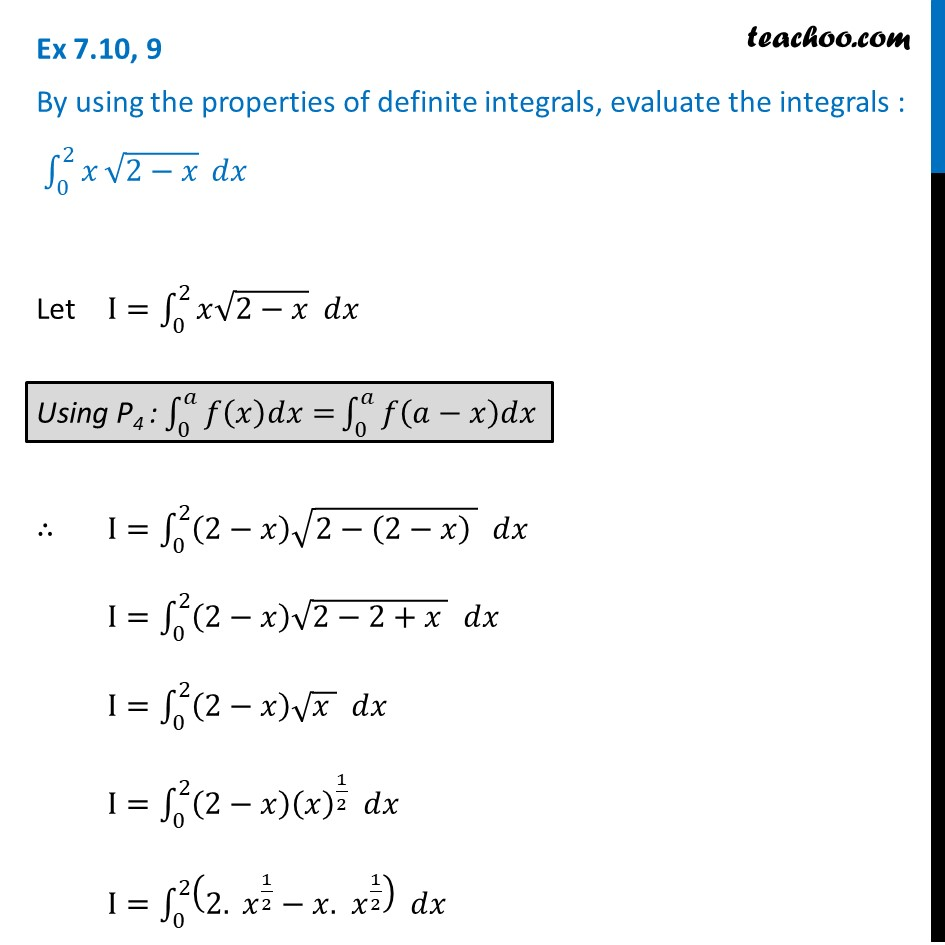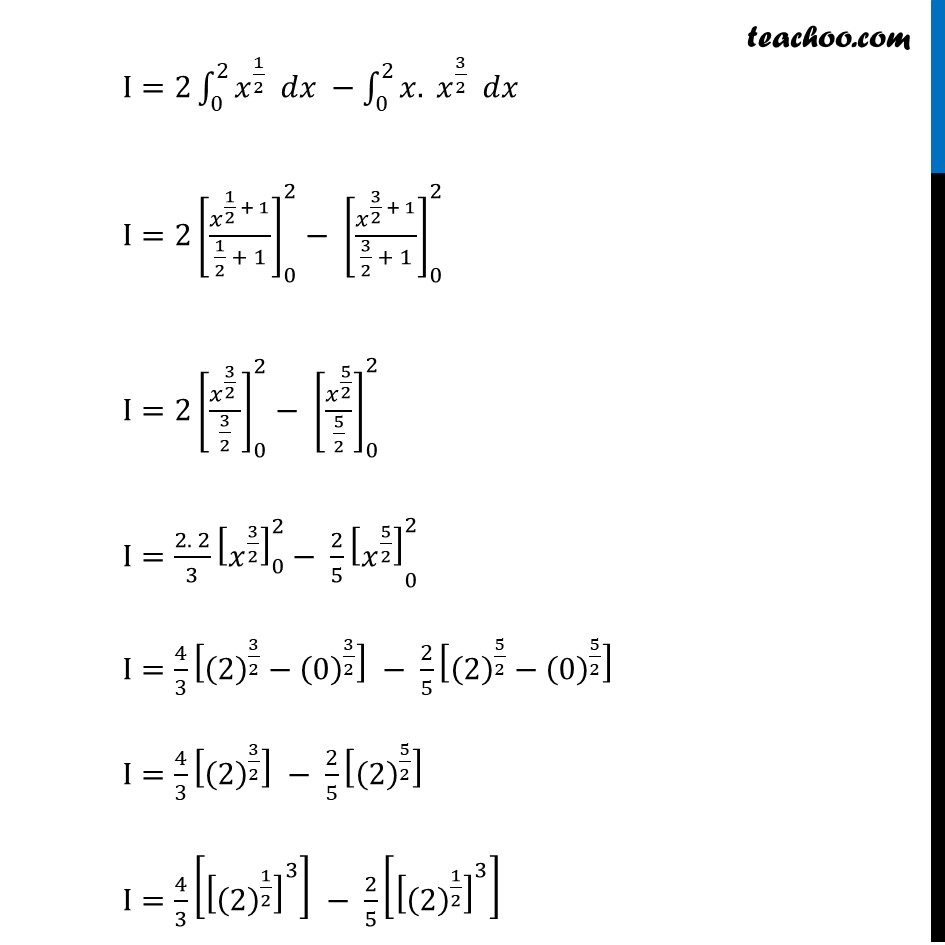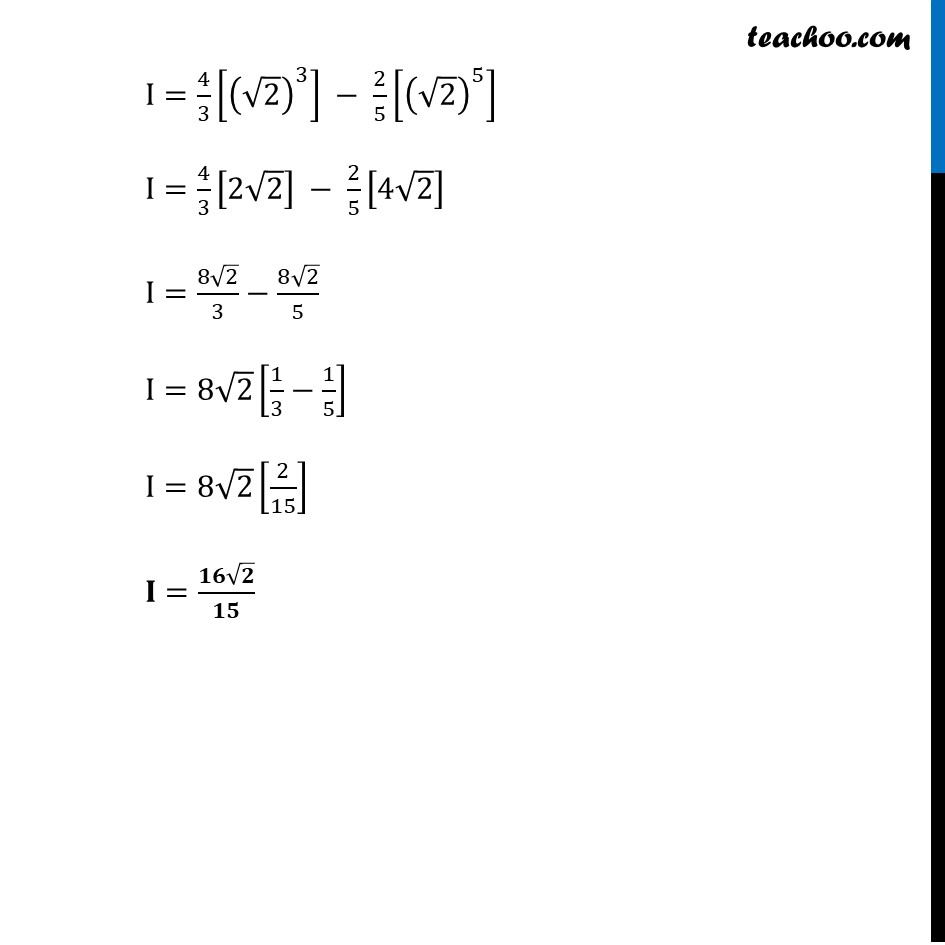Definite Integration by properties - P4

Chapter 7 Class 12 Integrals
Concept wiseLearn in your speed, with individual attention - Teachoo Maths 1-on-1 Class

### Transcript

Ex 7.10, 9 By using the properties of definite integrals, evaluate the integrals : ∫_0^2▒𝑥⁡√(2−𝑥) 𝑑𝑥 Let I=∫_0^2▒〖𝑥√(2−𝑥) 𝑑𝑥〗 ∴ I=∫_0^2▒〖(2−𝑥) √(2−(2−𝑥) ) 𝑑𝑥〗 I=∫_0^2▒〖(2−𝑥) √(2−2+𝑥 ) 𝑑𝑥〗 I=∫_0^2▒〖(2−𝑥) √(𝑥 ) 𝑑𝑥〗 I=∫_0^2▒〖(2−𝑥) (𝑥)^(1/2) 𝑑𝑥〗 I=∫_0^2▒〖(2. 𝑥^(1/2)−𝑥.〖 𝑥〗^(1/2) ) 𝑑𝑥〗 I=2∫_0^2▒〖𝑥^(1/2) 𝑑𝑥〗 −∫_0^2▒〖𝑥. 𝑥^(3/2) 𝑑𝑥〗 I=2[𝑥^(1/2 + 1)/(1/2 + 1)]_0^2− [𝑥^(3/2 + 1)/(3/2 + 1)]_0^2 I=2[𝑥^(3/2 )/(3/2)]_0^2− [𝑥^(5/2)/(5/2)]_0^2 I=(2. 2)/3 [𝑥^(3/2) ]_0^2− 〖2/5 [𝑥^(5/2) ]〗_0^2 I=4/3 [(2)^(3/2)−(0)^(3/2) ] − 2/5 [(2)^(5/2)−(0)^(5/2) ] I=4/3 [(2)^(3/2) ] − 2/5 [(2)^(5/2) ] I=4/3 [[(2)^(1/2) ]^3 ] − 2/5 [[(2)^(1/2) ]^3 ] I=4/3 [(√2)^3 ] − 2/5 [(√2)^5 ] I=4/3 [2√2] − 2/5 [4√2] I=(8√2)/3−(8√2)/5 I=8√2 [1/3−1/5] I=8√2 [2/15] 𝐈=(𝟏𝟔√𝟐)/𝟏𝟓